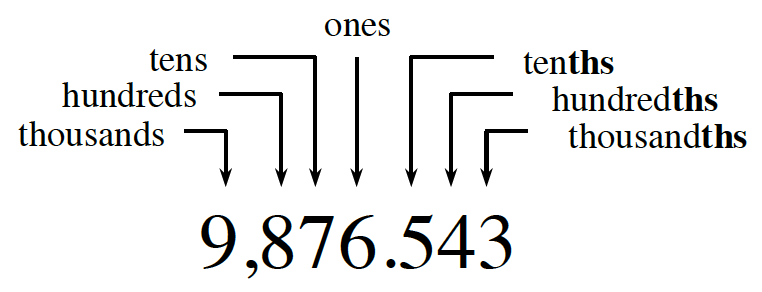### Home > CC1MN > Chapter 1 > Lesson 1.1.4 > Problem1-31

1-31.

Round each number to the specified place. Read the Math Notes box in this lesson for a reminder of rounding to a given place value.

1. $5,294.6$
(hundred)

•If you need help with place values, refer to this graphic from the Math Notes box in Lesson 1.1.3.

To start, find the digit in the place specified by the problem (here, the hundreds place).
$\text{(The hundred's place is the }2 \text{ in this #}\rightarrow\quad 5294.6 \text{)}$

Now, find the digit one place to the right. If it is greater than or equal to 5, increase the specified digit by 1. $\text{(The digit before the hundred's place is the }9 \text{ in this #} \rightarrow 5294.6\text{)}$

Replace all of the digits to the right of the specified one with zeros to finish.

$5300$

1. $45,469.23$
(thousand)

See part (a).

2. $7526.442$
(hundredth)

$7526.44$

3. $492.3069$
(thousandth)

See part (a).